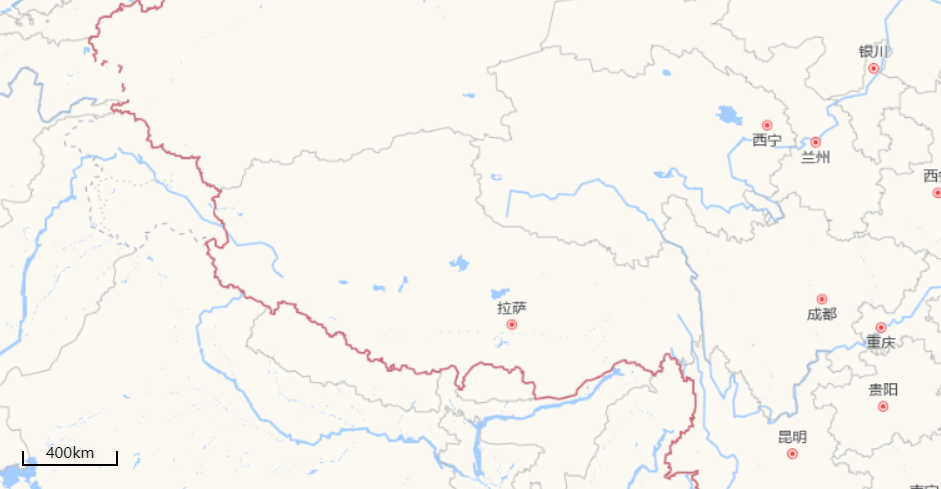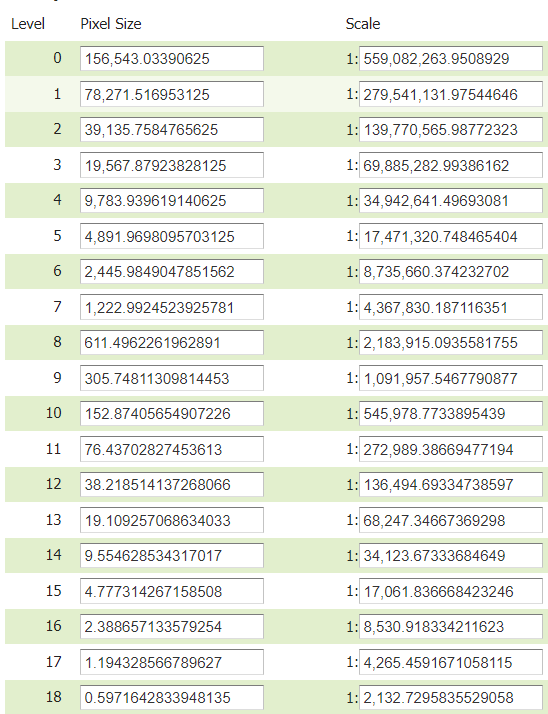2021/11/19 22:07

# 概述

比例尺在地图中是一个非常重要的概念，有着辅助读图的作用。本文在ol框架下，实现webgis中的比例尺功能。

# 实现效果# 概念# 实现

const minWidth = 60
const dom = document.getElementById('scaleLine')
let currentZoom = -1
// 地图拖动和缩放事件
map.on('moveend', function (e) {
if(currentZoom === map.getView().getZoom()) return
const res = map.getView().getResolution()
let width = minWidth;
let minScale = res * width
let unit = 'm', scale = minScale
if(minScale / 1000 > 1) {
unit = 'km'
scale = Math.ceil(minScale / 1000)
}
const breaks = [1000, 500, 200, 100, 50, 20, 10]
for (let i = 0; i < breaks.length; i++) {
const b = breaks[i]
if(scale / b > 1) {
scale = Math.ceil(scale / b) * b
break
}
}
width = unit === 'm' ? scale / res : scale * 1000 / res
dom.style.width = (width + 4) + 'px'
dom.innerText = scale + unit
currentZoom = map.getView().getZoom()
})


1. minWidth是展示比例尺最小的宽度，也是后面计算比例尺的一个基准；
2. 比例尺的展示是通过一个浮动的div来展示的；
3. 通过当前分辨率计算60个像素对应的图上的距离，并做取整处理；### 作者的其它热门文章

0
0 收藏

0 评论
0 收藏
0